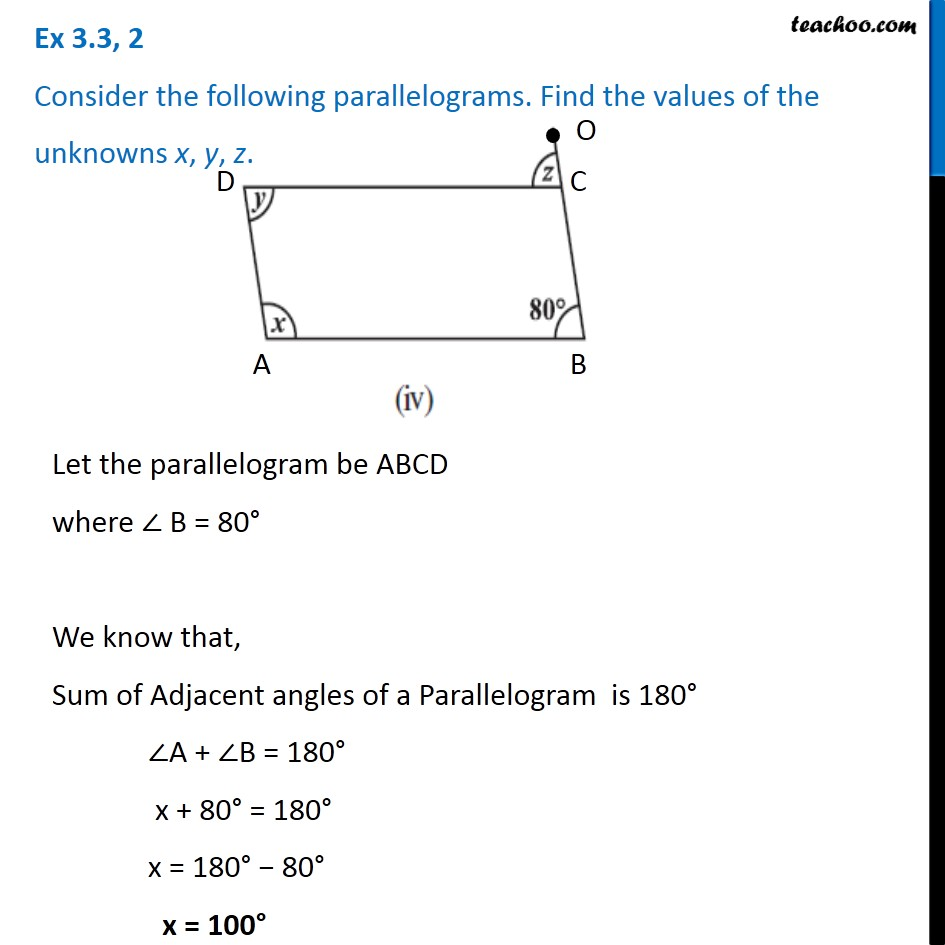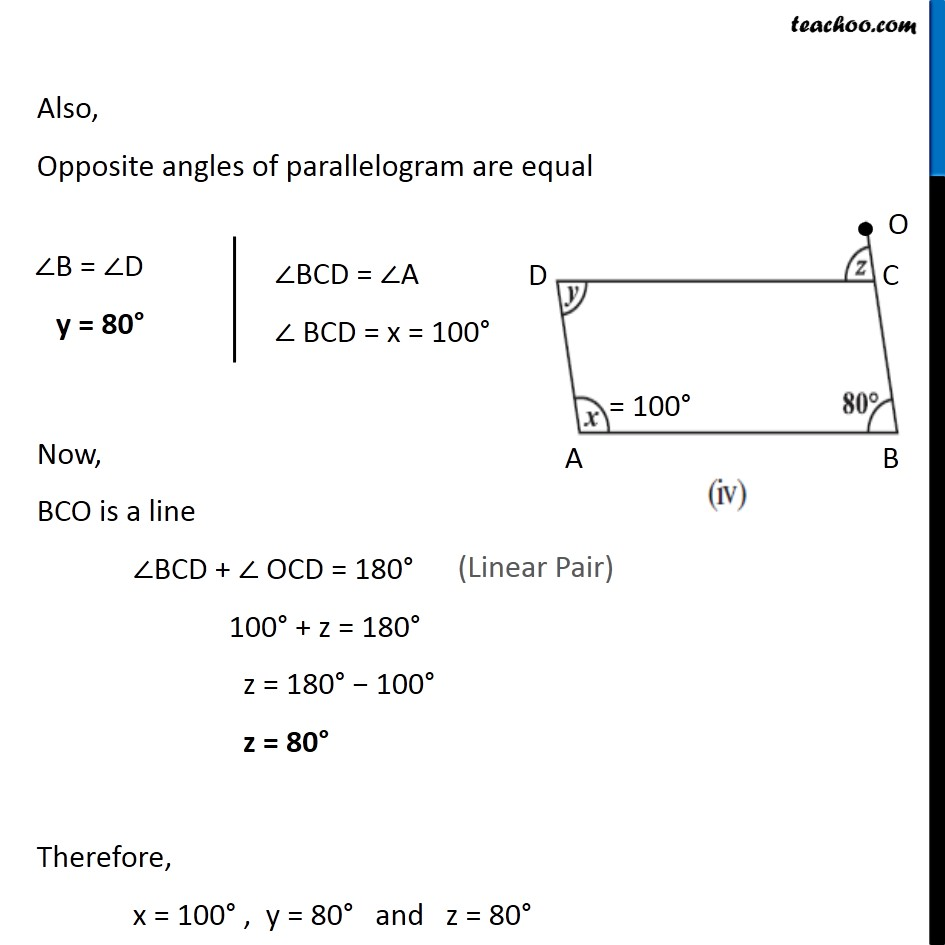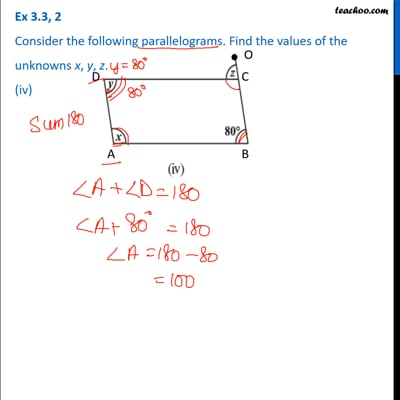Ex 3.3

Chapter 3 Class 8 Understanding Quadrilaterals
Serial order wiseThis video is only available for Teachoo black users

### Transcript

Ex 3.3, 2 Consider the following parallelograms. Find the values of the unknowns x, y, z. Let the parallelogram be ABCD where ∠ B = 80° We know that, Sum of Adjacent angles of a Parallelogram is 180° ∠A + ∠B = 180° x + 80° = 180° x = 180° − 80° x = 100° Also, Opposite angles of parallelogram are equal ∠B = ∠D y = 80° ∠BCD = ∠A ∠ BCD = x = 100°Now, BCO is a line ∠BCD + ∠ OCD = 180° 100° + z = 180° z = 180° − 100° z = 80° Therefore, x = 100° , y = 80° and z = 80°